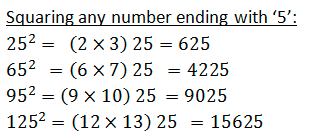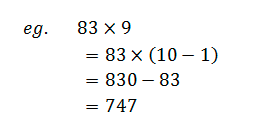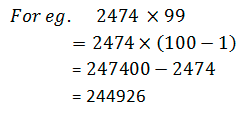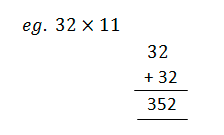# Crack JEE by Learning these 5 Maths TricksHaven’t you been amazed by those “Math magicians” who take less than a second to solve super complex equations?

And we just watch with our gaped mouths!

No, we are not expecting you to become one but we thought of sharing 5 Maths tricks that will help you calculate faster

Imagine that for an exam like JEE, one of the toughest in the country, how helpful these tricks would be. It will ensure faster calculations and hence less time. And when you have some spare time, why not spend it to fetch some more marks, may be!

• Squaring any number ending with 5

Write down the square of 5 in the place of last 2 digits and multiply the rest of the number with its consecutive number to get the correct result. Have a look at the below examples:• Multiplication of any 3-digit numbers

It might look bit complex at the start but once you practice some multiplications, it is very beneficial to yield accurate results faster.

Take any two numbers like 208 and 206
Now subtract the number at units place
208-8=200
206-6=200
Now select any number and add the unit digit of another number
208+6=214
Now multiply, 214×200=42800
Now multiply the unit digits of both numbers, 8×6=48
The product of the numbers 208 and 206 is 42848

• Squaring 2-digit numbers

This is quite a common and time taking calculation in general but not once you master the below trick

Take any number such as 77. Now add or subtract the number to make it to its nearest multiple of 10.
In this case, add 3 to the number to reach the nearest 10, i.e., 77+3=80
Now, (77+3) x (77-3) =80 x 74=5920
The number add was 3. Now square the number and add it to the above product.
Square of 3=9
5920+9=5929

• Multiplying a number by 9 and 11

Multiplying By 9: Keep the base number same and split the ‘9’ number into 10 or its multiples as shown below. Multiplication by a multiple of 10 is much easier to get the result faster.Multiplying by 11: Shift the number by one unit and add to the same number.• Binary and bisect

This is the best one! If you need to find a product of two numbers at least one of which is even, keep on doubling one number and halving the even number till you reach the simpler calculation:

For example, instead of multiplying 63*24, you can do 126*12, again 252*6, and further 504*3 = 1512.

Every small step you take towards JEE preparation makes it more efficient. Learn and use these easy tricks to attain an edge over your competitors.

Check Your Skills With : Free JEE Mock Test

What are you waiting for?